math tutorials >quadratic equations math review

## In a quadratic equation the highest power a variable has is 2.

So in the examples below, x has a power of 2. We can also say that x is squared.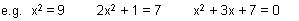## Simple quadratic equations are solved in the same way as linear equations.

First rearrange the equation to get variables on one side and numbers on the other.  Then perform the same operation on both sides of the equation until you have solved for x.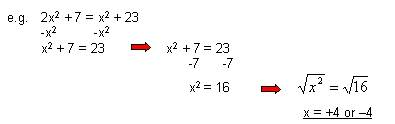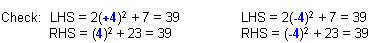You will notice that quadratic equations usually have 2 possible solutions (often a positive and a negative solution like in the examples above).

## Solving more difficult quadratic equations.

If 2 numbers multiplied together give zero, then either one number must be zero, or both must be zero.

e.g. If ab = 0, then either a = 0 or b = 0 or a = b = 0 (ie they are both equal to 0).

We use this information to solve factorised quadratic equations.

1. Rearrange the equation to make it equal to zero.
2. Factorise the equation.
3. Solve for x.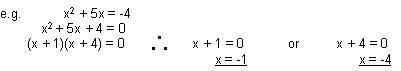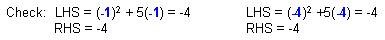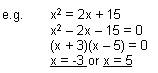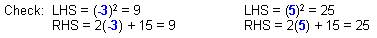## Algebra problems for you to try.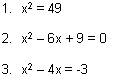Solutions:  1)  x = 7 or –7          2)  x = 3            3) x = 3 or 1